# Co(ll) was used as an internal standard to analyze a sample of Ti(IV) with atomic absorption spectroscopy (AAS). A standard mixture containing 1.90 g Co/mL and 1.53 /g Ti/mL measured by AAS produced...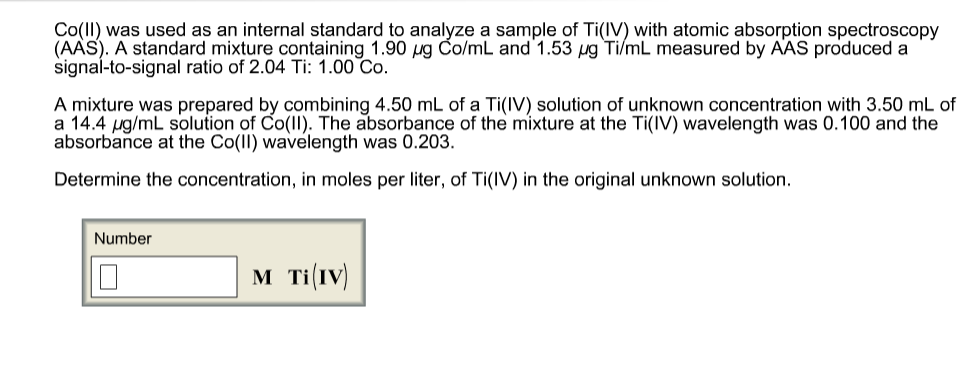Co(ll) was used as an internal standard to analyze a sample of Ti(IV) with atomic absorption spectroscopy (AAS). A standard mixture containing 1.90 g Co/mL and 1.53 /g Ti/mL measured by AAS produced a signal-to-signal ratio of 2.04 Ti: 1.00 Co A mixture was prepared by combining 4.50 mL of a Ti(IV) solution of unknown concentration with 3.50 mL of a 14.4 ug/mL solution of Co(l1). The absorbance of the mixture at the Ti(IV) wavelength was 0.100 and the absorbance at the Co(Il) wavelength was 0.203 Determine the concentration, in moles per liter, of Ti(IV) in the original unknown solution Number M TiIV)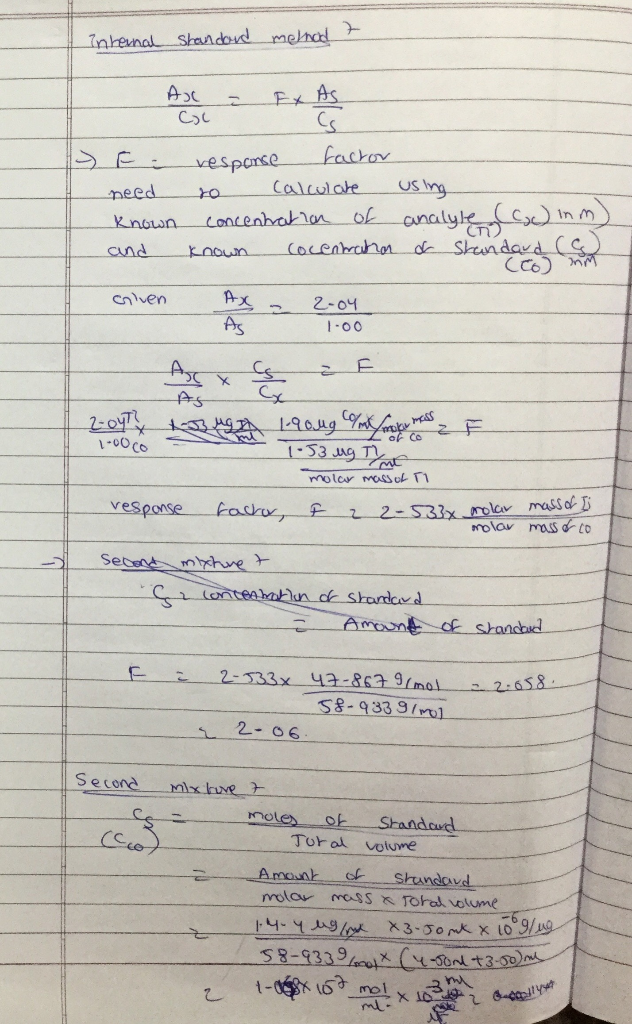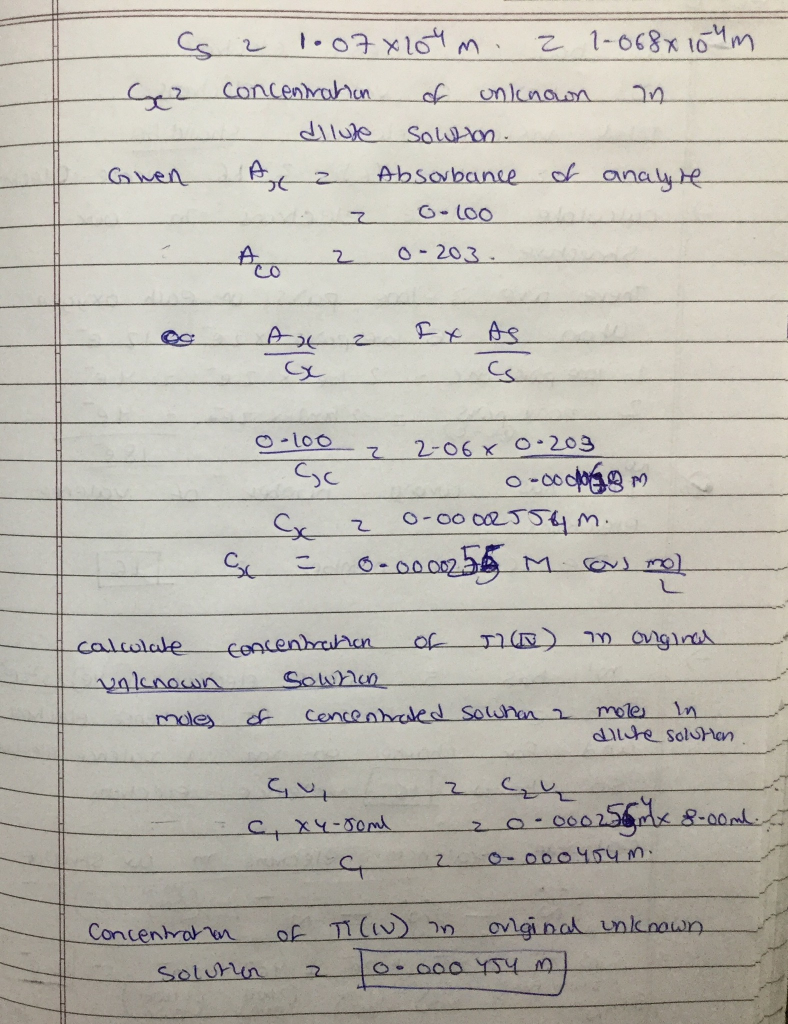##### Add Answer of: Co(ll) was used as an internal standard to analyze a sample of Ti(IV) with atomic absorption spectroscopy (AAS). A standard mixture containing 1.90 g Co/mL and 1.53 /g Ti/mL measured by AAS produced...
Similar Homework Help Questions
• ### Mn was used as an internal standard for measuring Fe by atomic absorption. A standard mixture...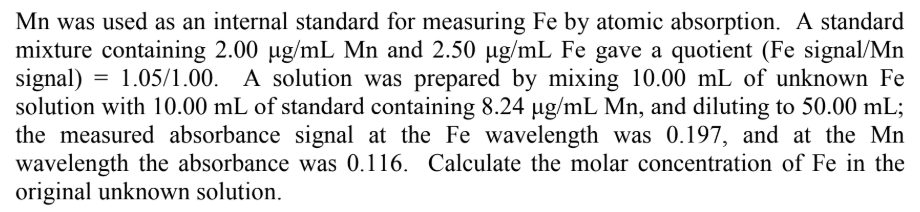Mn was used as an internal standard for measuring Fe by atomic absorption. A standard mixture containing 2.00 Hg/mL Mn and 2.50 Hg/mL Fe gave a quotient (Fe signal/Mn signal) 1.05/1.00. A solution was prepared by mixing 10.00 mL of unknown Fe solution with 10.00 mL of standard containing 8.24 ug/mL Mn, and diluting to 50.00 mL; the measured absorbance signal at the Fe wavelength was 0.197, and at the Mn wavelength the absorbance was 0.116. Calculate the molar concentration...

• ### Quantitative Chemical Analysis

Mn was used as an internal standard for measuring Fe by atomic absorption spectroscopy. A standard mixture containing 2.00 ppm and 2.50 ppm gave a Fe / Mn signal ratio(quotient) of 1.05. A 5.00 mL unknown Fe solution was mixed with 1.00 mL solution containing 12.5 ppm Mn. The absorbance of this mixture at the Mn wavelength was 0.128and the absorbance at the Fe wavelength was 0.128. Find the concentration of the unknown Fe solution. (Note: In AAS each element...

• ### Atomic absorption spectroscopy was used with the method of standard additions to determine the concentration of cadmium...

Atomic absorption spectroscopy was used with the method of standard additions to determine the concentration of cadmium in a sample of an industrial waste stream. For the addition, 10.0 μL of a 1000.0 μg/mL Cd standard was added to 10.0 mL of the unknown solution. The results obtained are shown in the table. Absorbance Reagent blank 0.023 Sample 0.498 Sample plus addition 0.714 What is the concentration of the cadmium in the waste stream sample?

• ### Absorbance

2. Mn was used as an internal standard for measuring Fe by atomic absorption spectroscopy. A standard mixture containing 2.00 ppm and 2.50 ppm gave a Fe / Mn signalratio (quotient) of 1.05. A 5.00 mL unknown Fe solution was mixed with 1.00 mL solution containing 12.5 ppm Mn. The absorbance of this mixture at the Mn wavelength was0.128 and the absorbance at the Fe wavelength was 0.128. Find the concentration of the unknown Fe solution. (Note: In AAS each...

• ### Atomic absorption spectroscopy was used with the method of standard additions to determine the concentration of cadmium...

Atomic absorption spectroscopy was used with the method of standard additions to determine the concentration of cadmium in a sample of an industrial waste stream. For the addition, 10.010.0 μL of a 1000.0 μg/mL CdCd standard was added to 10.0 mL of the unknown solution. The results obtained are shown in the table. Reagent blank 0.023 Sample 0.342 Sample plus addition 0.749 What is the concentration of the cadmium in the waste stream sample? Later, the analyst learned that the...

• ### Copper was determined in a river water sample by atomic absorption spectrometry and the method of...

Copper was determined in a river water sample by atomic absorption spectrometry and the method of standard additions. For the addition, 250.0 μL of a 1000.0-μg/mL standard was added to 150.0 mL of solution. The following data were obtained Absorbance of reagent blank = 0.023 Absorbance of sample = 0.519 Absorbance of sample plus addition - blank = 1.017 Calculate the copper concentration in the sample. Later studies showed that the reagent blank used to obtain the above data was...

• ### please show steps. A solution was prepared by mixing 10.00 mL of unknown (X) with 5.00 mL of standard (S) containing...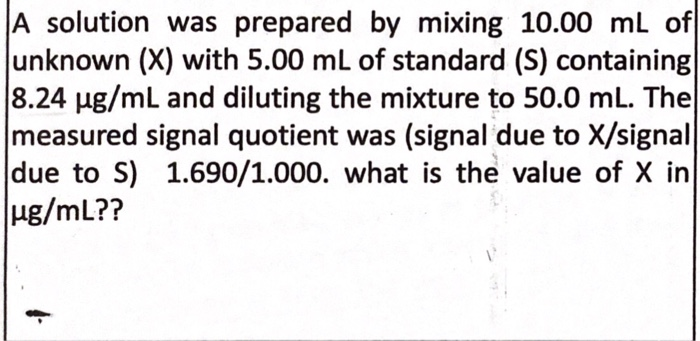please show steps. A solution was prepared by mixing 10.00 mL of unknown (X) with 5.00 mL of standard (S) containing 8.24 μg/mL and diluting the mixture to 50.0 mL. The measured signal quotient was (signal due to X/signal due to S) 1.690/1.000. what is the value of X in A solution was prepared by mixing 10.00 mL of unknown (X) with 5.00 mL of standard (S) containing 8.24 μg/mL and diluting the mixture to 50.0 mL. The measured signal...

• ### A. 0.191 g of steel was dissolved in acid and diluted to 100 mL with water. The solution was found to contain 7.7 ppm Mn by atomic absorption analysis. What was the weight percent Mn in the steel? B....

A. 0.191 g of steel was dissolved in acid and diluted to 100 mL with water. The solution was found to contain 7.7 ppm Mn by atomic absorption analysis. What was the weight percent Mn in the steel? B. A multivitamin tablet with a label value of 13 mg Zn per tablet was dissolved in acid and diluted to 100 mL with water. What is the theoretical Zn concentration of the solution? Answer in ppm.

Need Online Homework Help?